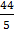# Two identical spherical balls of mass M and radius R each are stuck on two ends of a rod of length 2R and mass M (see figure). The moment of inertia of the system about the axis passing perpendicularly through the centre of the rod is:(a)MR2 (b)MR2 (c)MR2 (d)MR2

## Question ID - 50287 :- Two identical spherical balls of mass M and radius R each are stuck on two ends of a rod of length 2R and mass M (see figure). The moment of inertia of the system about the axis passing perpendicularly through the centre of the rod is:(a)MR2 (b)MR2 (c)MR2 (d)MR2

3537

For Ball

Using Parallel axis theorem.

Iball =MR2+M(2R)2

=MR2

2 Balls  soMR2

Irod =for rod=Isystem= IBall + Irod

=MR2+=MR2

Next Question :
 A body is projected at t=0 with a velocity 10 ms−1 at an angle of 60° with the horizontal. The radius of its trajectory at t=1s is R. Neglecting air resistance and taking acceleration due to gravity g=10 ms−2, the value of R is: (a) 2.5 m (b) 10.3 m (c) 2.8 m (d) 5.1 m